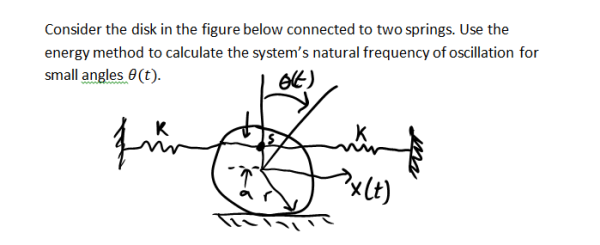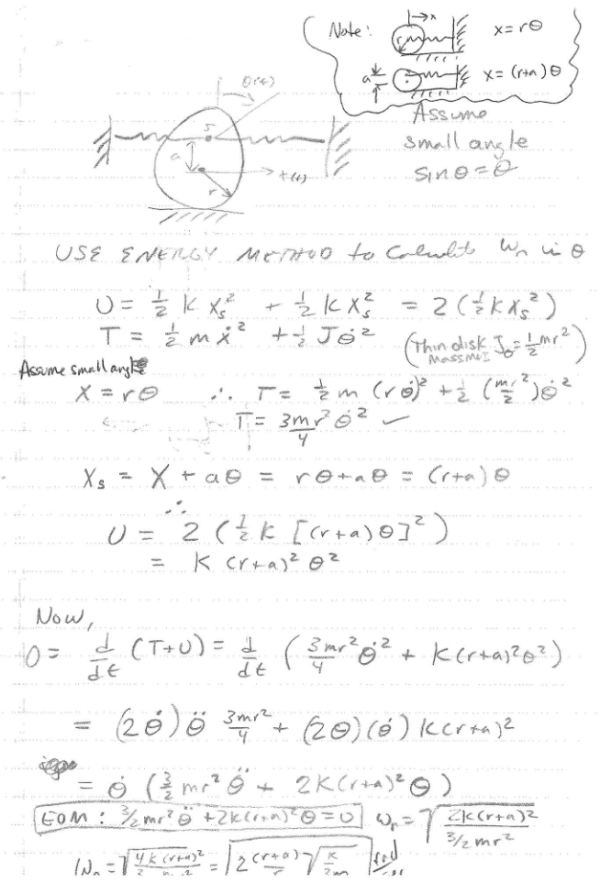Consider the disk in the figure below connected to two springs. Use the energy method to calculate the system's natural frequency of oscillation for small angles theta(t).Consider the disk in the figure below connected to two springs. Use the energy method to calculate the system's natural frequency of oscillation for small angles theta(t).

Vibrations Page 1 vibrations vibrations vibrations vibrations vibrations vibrations vibrations Vibrations Page 2